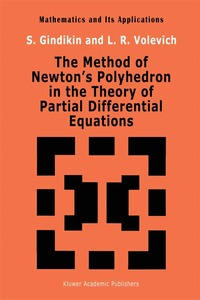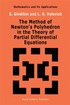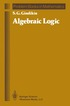Fler böcker inom
Format
Inbunden (Hardback)
Språk
Engelska
Antal sidor
266
Utgivningsdatum
1992-11-01
Upplaga
1992 ed.
Förlag
Springer
Översättare
V M Volosov
Originalspråk
Russian
Medarbetare
Volevich, L.R.
Illustratör/Fotograf
references index
Illustrationer
X, 266 p.
Dimensioner
243 x 165 x 22 mm
Vikt
581 g
Antal komponenter
1
Komponenter
1 Hardback
ISBN
9780792320371

### Du kanske gillar# The Method of Newton's Polyhedron in the Theory of Partial Differential Equations

Inbunden Engelska, 1992-11-01
1099
• Skickas inom 10-15 vardagar.
• Gratis frakt inom Sverige över 159 kr för privatpersoner.
Finns även som
909
• Skickas inom 10-15 vardagar
1009
One service mathematics has rendered the 'Et moi, ..., si j'avait su comment cn rcvenir, human race. It has put common sense back. je n'y serais point aile.' where it bdongs, on the topmost shelf neAt Jules Verne to the dusty canister labelled 'discarded non* sense'. The series is divergent; therefore we may be Eric T. Bdl able to do something with it. O. Heaviside Mathematics is a tool for thought. A highly necessary tool in a world where both feedback and non- linearities abound. Similarly, all kinds of parts of mathematics serve as tools for other parts and for other sciences. Applying a simple rewriting rule to the quote on the right above one finds such statements as: 'One service topology has rendered mathematical physics ...'; 'One service logic has rendered com- puter science .. :; 'One service category theory has rendered mathematics ...'. All a,rguably true. And all statements obtainable this way form part of the raison d'etre of this series.

## Passar bra ihop

1.2. +
3.De som köpt den här boken har ofta också köpt Algebraic Logic av Semen G Gindikin (häftad).

## Kundrecensioner

Har du läst boken? Sätt ditt betyg »

## Innehållsförteckning

1. Two-sided estimates for polynomials related to Newton's polygon and their application to studying local properties of partial differential operators in two variables.- 1. Newton's polygon of a polynomial in two variables.- 2. Polynomials admitting of two-sided estimates.- 3. N Quasi-elliptic polynomials in two variables.- 4. N Quasi-elliptic differential operators.- Appendix to 4.- 2. Parabolic operators associated with Newton's polygon.- 1. Polynomials correct in Petrovski?'s sense.- 2. Two-sided estimates for polynomials in two variables satisfying Petrovski?'s condition. N-parabolic polynomials.- 3. Cauchy's problem for N-stable correct and N-parabolic differential operators in the case of one spatial variable.- 4. Stable-correct and parabolic polynomials in several variables.- 5. Cauchy's problem for stable-correct differential operators with variable coefficients.- 3. Dominantly correct operators.- 1. Strictly hyperbolic operators.- 2. Dominantly correct polynomials in two variables.- 3. Dominantly correct differential operators with variable coefficients (the case of two variables).- 4. Dominantly correct polynomials and the corresponding differential operators (the case of several spatial variables).- 4. Operators of principal type associated with Newton's polygon.- 1. Introduction. Operators of principal and quasi-principal type.- 2. Polynomials of N-principal type.- 3. The main L2 estimate for operators of N-principal type.- Appendix to 3.- 4. Local solvability of differential operators of N-principal type.- Appendix to 4.- 5. Two-sided estimates in several variables relating to Newton's polyhedra.- 1. Estimates for polynomials in ?n relating to Newton's polyhedra.- 2. Two-sided estimates in some regions in ?n relating to Newton's polyhedron. Special classes of polynomials and differential operators in several variables.- 6. Operators of principal type associated with Newton's polyhedron.- 1. Polynomials of N-principal type.- 2. Estimates for polynomials of N-principal type in regions of special form.- 3. The covering of ?n by special regions associated with Newton's polyhedron.- 4. Differential operators of ?n-principal type with variable coefficients.- Appendix to 4.- 7. The method of energy estimates in Cauchy's problem 1. Introduction. The functional scheme of the proof of the solvability of Cauchy's problem.- 2. Sufficient conditions for the existence of energy estimates.- 3. An analysis of conditions for the existence of energy estimates.- 4. Cauchy's problem for dominantly correct differential operators.- References.